• ## 深度优先遍历和广度优先遍历

万次阅读 多人点赞 2019-05-29 21:24:38
深度优先遍历简称DFS（Depth First Search），广度优先遍历简称BFS（Breadth First Search），它们是遍历图当中所有顶点的两种方式。 这两种遍历方式有什么不同呢？我们来举个栗子： 我们来到一个游乐场，游乐场里有...
深度优先遍历和广度优先遍历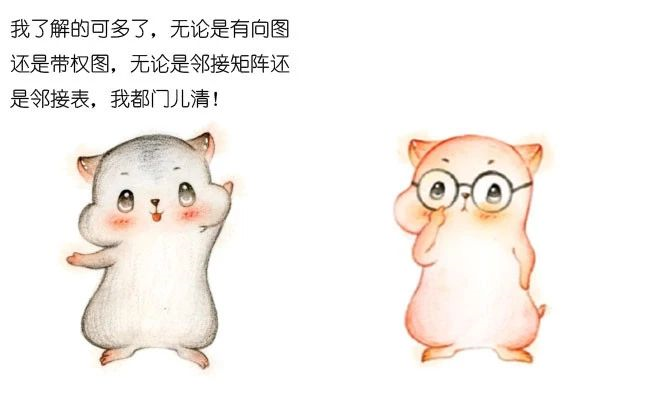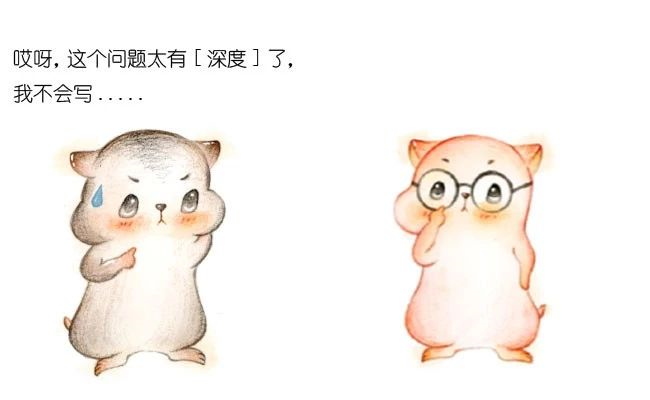什么是 深度/广度 优先遍历？
这两种遍历方式有什么不同呢？我们来举个栗子：
我们来到一个游乐场，游乐场里有11个景点。我们从景点0开始，要玩遍游乐场的所有景点，可以有什么样的游玩次序呢？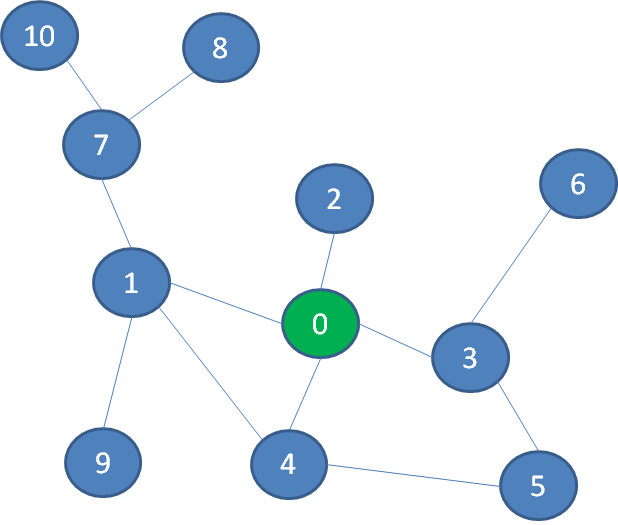第一种是一头扎到底的玩法。我们选择一条支路，尽可能不断地深入，如果遇到死路就往回退，回退过程中如果遇到没探索过的支路，就进入该支路继续深入。
在图中，我们首先选择景点1的这条路，继续深入到景点7、景点8，终于发现走不动了（景点旁边的数字代表探索次序）：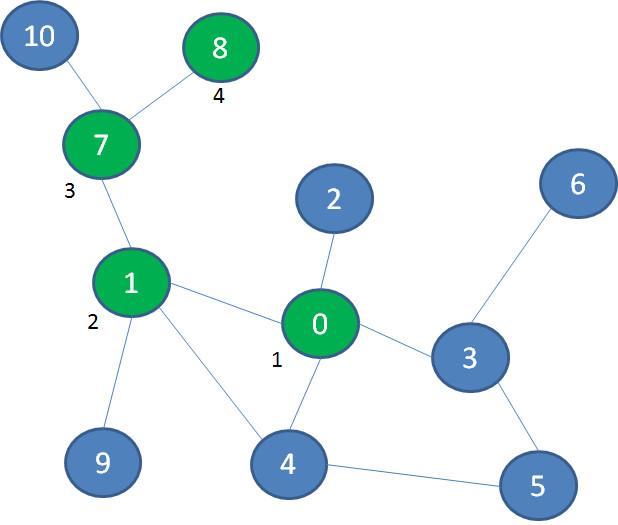于是，我们退回到景点7，然后探索景点10，又走到了死胡同。于是，退回到景点1，探索景点9：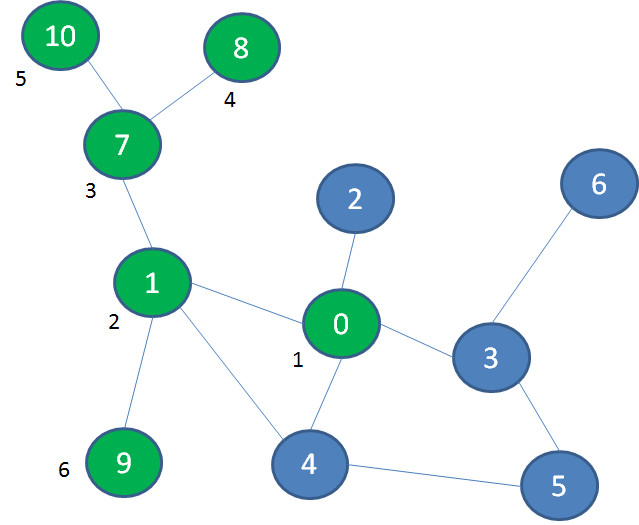按照这个思路，我们再退回到景点0，后续依次探索景点2、3、5、4、6，终于玩遍了整个游乐场：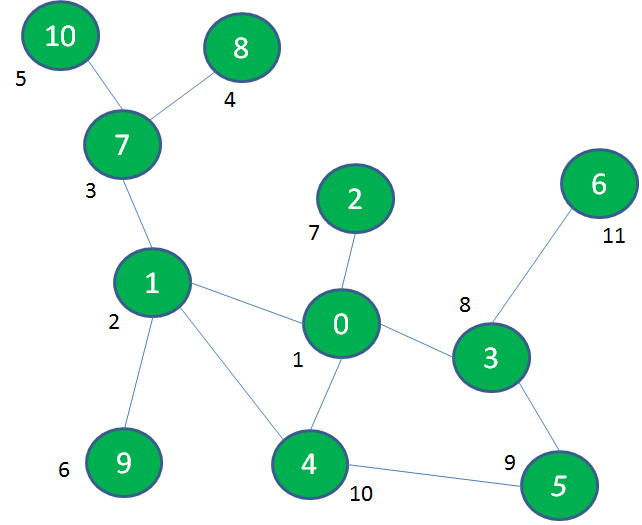像这样先深入探索，走到头再回退寻找其他出路的遍历方式，就叫做深度优先遍历（DFS）。除了像深度优先遍历这样一头扎到底的玩法以外，我们还有另一种玩法：首先把起点相邻的几个景点玩遍，然后去玩距离起点稍远一些（隔一层）的景点，然后再去玩距离起点更远一些（隔两层）的景点…
在图中，我们首先探索景点0的相邻景点1、2、3、4：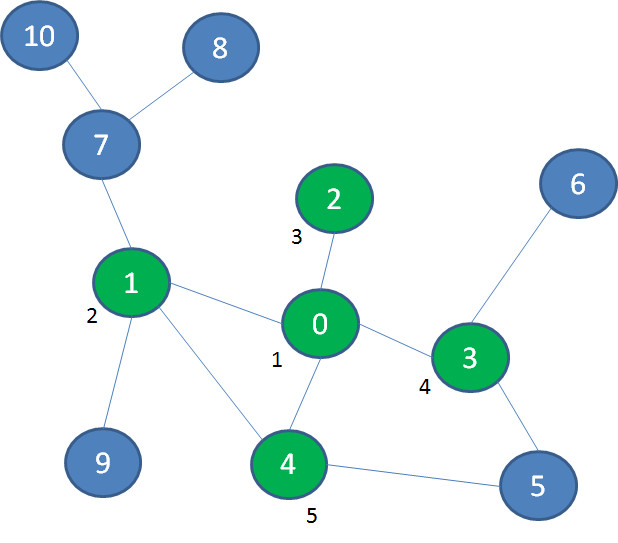接着，我们探索与景点0相隔一层的景点7、9、5、6：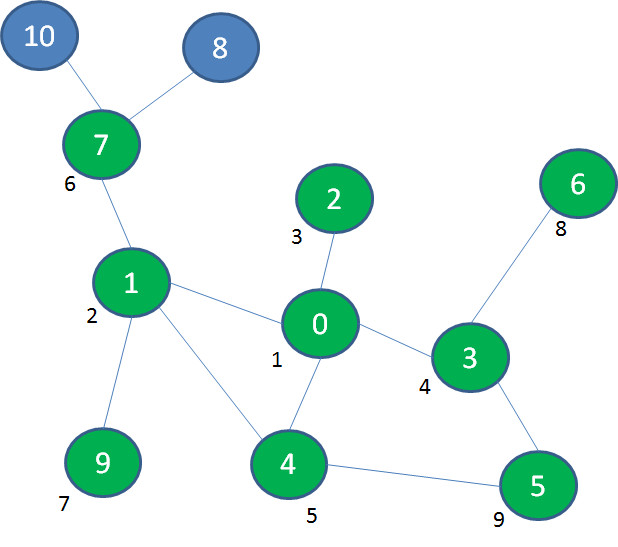最后，我们探索与景点0相隔两层的景点8、10：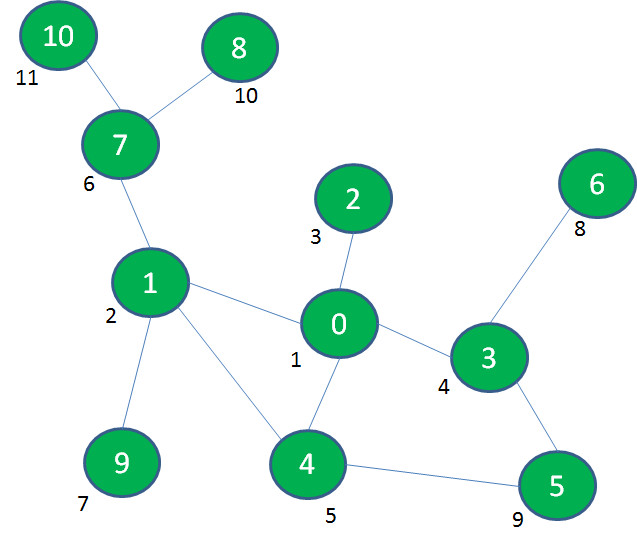像这样一层一层由内而外的遍历方式，就叫做广度优先遍历（BFS）。深度优先遍历
首先说说深度优先遍历的实现过程。这里所说的回溯是什么意思呢？回溯顾名思义，就是自后向前，追溯曾经走过的路径。
我们把刚才游乐场的例子抽象成数据结构的图，假如我们依次访问了顶点0、1、7、8，发现无路可走了，这时候我们要从顶点8退回到顶点7。而后我们探索了顶点10，又无路可走了，这时候我们要从顶点10退回到顶点7，再退回到顶点1。像这样的自后向前追溯曾经访问过的路径，就叫做回溯。
要想实现回溯，可以利用栈的先入后出特性，也可以采用递归的方式（因为递归本身就是基于方法调用栈来实现）。
下面我们来演示一下具体实现过程。
首先访问顶点0、1、7、8，这四个顶点依次入栈，此时顶点8是栈顶：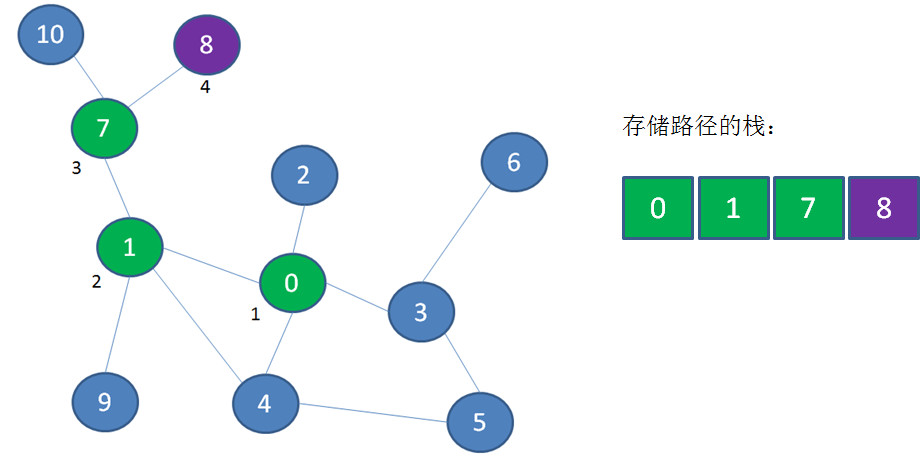从顶点8退回到顶点7，顶点8出栈：接下来访问顶点10，顶点10入栈：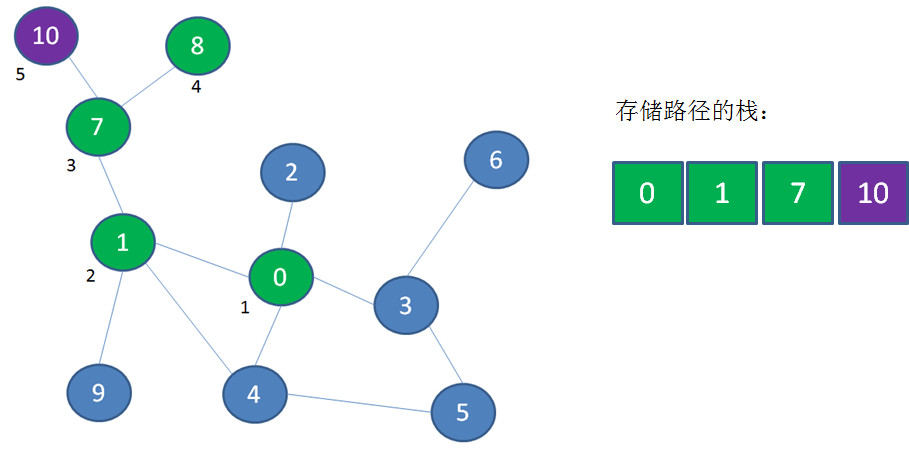从顶点10退到顶点7，从顶点7退到顶点1，顶点10和顶点7出栈：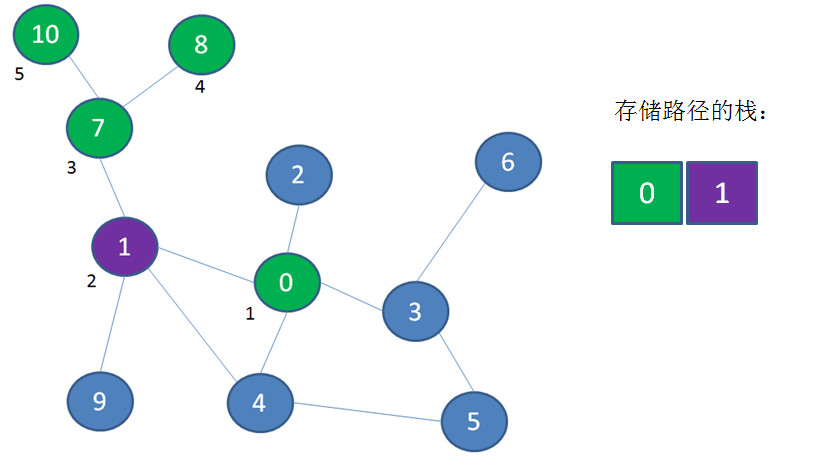探索顶点9，顶点9入栈：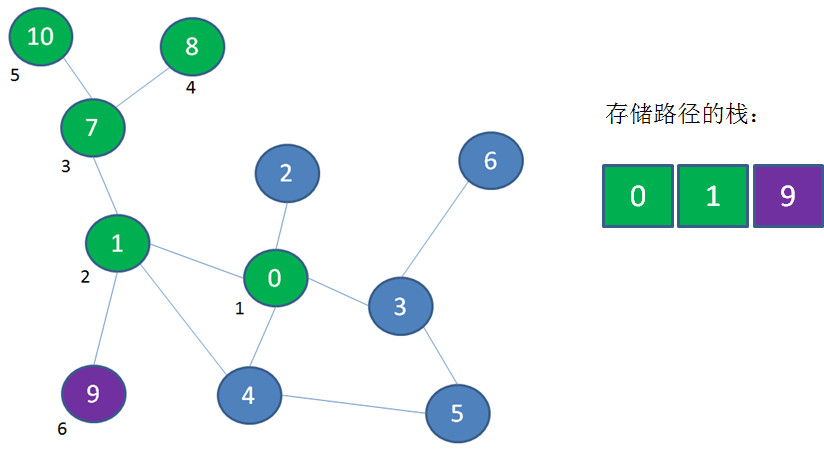以此类推，利用这样一个临时栈来实现回溯，最终遍历完所有顶点。
广度优先遍历
接下来该说说广度优先遍历的实现过程了。刚才所说的重放是什么意思呢？似乎听起来和回溯差不多？其实，回溯与重放是完全相反的过程。
仍然以刚才的图为例，按照广度优先遍历的思想，我们首先遍历顶点0，然后遍历了邻近顶点1、2、3、4：接下来我们要遍历更外围的顶点，可是如何找到这些更外围的顶点呢？我们需要把刚才遍历过的顶点1、2、3、4按顺序重新回顾一遍，从顶点1发现邻近的顶点7、9；从顶点3发现邻近的顶点5、6。像这样把遍历过的顶点按照之前的遍历顺序重新回顾，就叫做重放。同样的，要实现重放也需要额外的存储空间，可以利用队列的先入先出特性来实现。
下面我们来演示一下具体实现过程。
首先遍历起点顶点0，顶点0入队：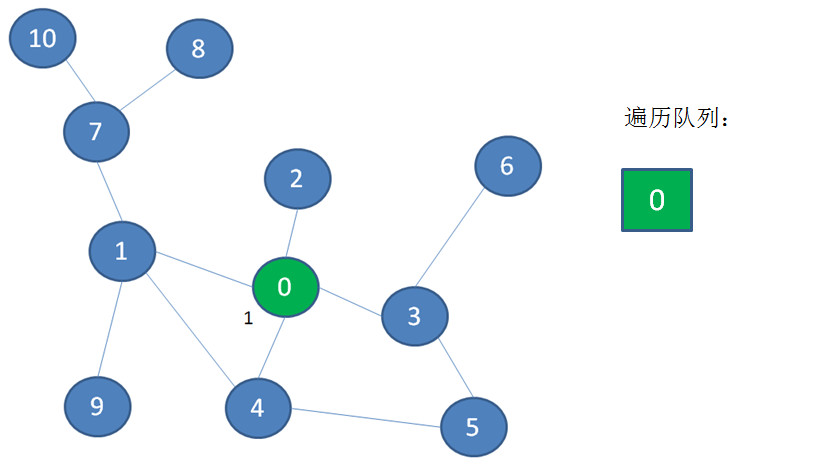接下来顶点0出队，遍历顶点0的邻近顶点1、2、3、4，并且把它们入队：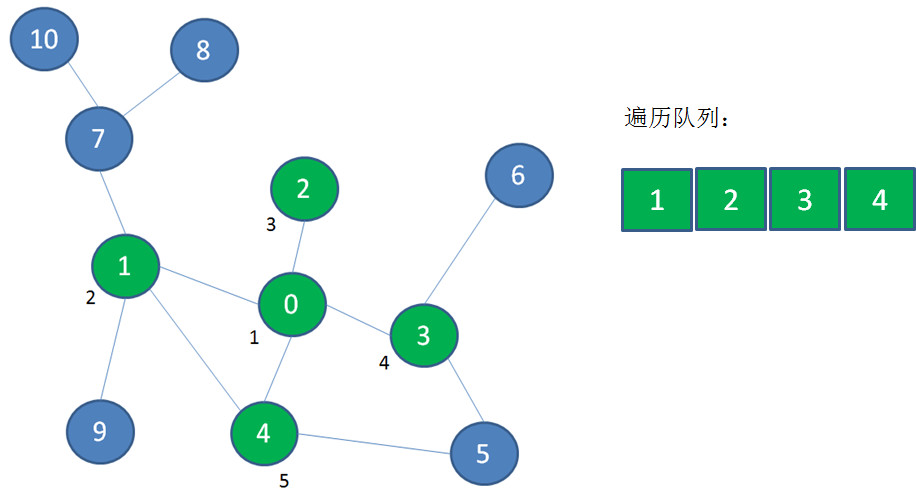然后顶点1出队，遍历顶点1的邻近顶点7、9，并且把它们入队：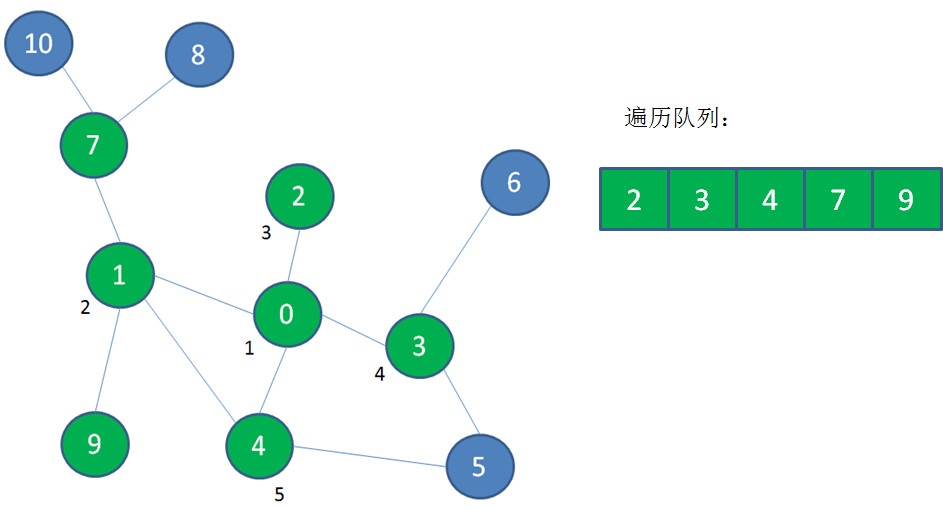然后顶点2出队，没有新的顶点可入队：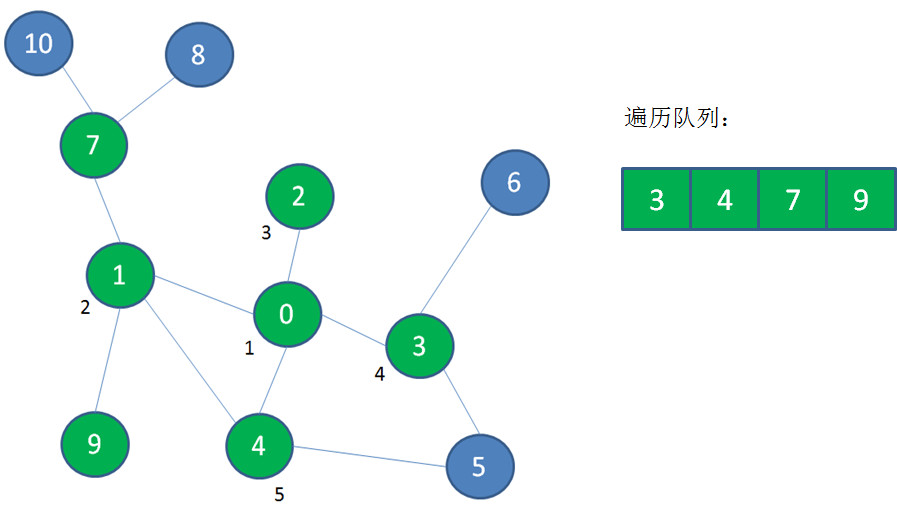以此类推，利用这样一个队列来实现重放，最终遍历完所有顶点。/**
图的顶点

/ private static class Vertex { int data; Vertex(int data) {    this.data = data; } } /*

图（邻接表形式）

/ private static class Graph { private int size; private Vertex[] vertexes; private LinkedList adj[]; Graph(int size){    this.size = size;    //初始化顶点和邻接矩阵    vertexes = new Vertex[size];    adj = new LinkedList[size];    for(int i=0; i*

深度优先遍历

/ public static void dfs(Graph graph, int start, boolean[] visited) { System.out.println(graph.vertexes[start].data); visited[start] = true; for(int index : graph.adj[start]){    if(!visited[index]){        dfs(graph, index, visited);    } } } /*

广度优先遍历

*/

public static void bfs(Graph graph, int start, boolean[] visited, LinkedList queue) {

queue.offer(start);

while (!queue.isEmpty()){

int front = queue.poll();

if(visited[front]){

continue;

}

System.out.println(graph.vertexes[front].data);

visited[front] = true;

queue.offer(index);;

}

}

}

public static void main(String[] args) {

Graph graph = new Graph(6);

System.out.println("图的深度优先遍历：");

dfs(graph, 0, new boolean[graph.size]);

System.out.println("图的广度优先遍历：");

bfs(graph, 0, new boolean[graph.size], new LinkedList());

}展开全文• 图深度优先遍历 void dfs(int i)//用数组模拟邻接表存储 ，访问点i { visited[i]=true; //标记为已访问过 for(int j=1;j<=num[i];j++) //遍历与i相关联的所有为访问过的顶点 if(!visited[g[i][j]]) dfs...
图深度优先遍历
void dfs(int i)//图用数组模拟邻接表存储  ，访问点i
{
visited[i]=true; //标记为已访问过
for(int j=1;j<=num[i];j++)  //遍历与i相关联的所有为访问过的顶点
if(!visited[g[i][j]])
dfs(g[i][j]);
}
int main()
{

……
memset(visited,false,sizeof(visited)) ;
for(int i=1;i<=n;i++)//每一个点都作为起点尝试访问 因为不是从任何一点开始都能遍历整个图
if(!visited[i])
dfs(i);

return 0;
}



展开全文• 深度优先遍历 广度优先遍历 图解算法过程
图的邻接矩阵表示
通常图的表示有两种方法：邻接矩阵，邻接表。
本文用邻接矩阵实现，一是代码量更少，二是代码风格也更贴近C语言。但不论是图的哪种实现方式，其基本的实现思想是不变的。
1：节点的信息，我们用一维数组a[n]来存储，假设图共有n个节点。
2：节点与节点间的关系，我们用二维数组b[n][n]存储。
3：b[i][j]表示，从i到j有向连通，b[j][i]表示从j到i有向连通，而当i=j时(矩阵的对角线上的元素)，b[i][j]没有实际意思，在遍历时，我可以用来存储定节点是否访问过。

图的深度优先遍历
DFS类似于树的先根遍历，尽可能深的去访问节点。
DFS算法过程我们用到了递归的思想，如果还需要求出路径，可以借助栈来实现(后文会不断的实现图的各种算法)。
步骤1：访问节点，将该节点标记为已访问。
步骤2：如果节点还有未标记的邻接点，继续步骤1，否则返回。

图的广度优先遍历
BFS我们用队列来实现。
步骤1：如果队列为空，则返回，否则，取出队列头节点，将该节点标记为已访问。
步骤2：同时，将该节点所有未标记的邻接点，加入队列尾，继续步骤1。

图的非递归遍历
我们借助栈来实现。
步骤1：如果栈为空，则退出程序，否则，访问栈顶节点，但不弹出栈点节点。
步骤2：如果栈顶节点的所有直接邻接点都已访问过，则弹出栈顶节点，否则，将该栈顶节点的未访问的其中一个邻接点压入栈，同时，标记该邻接点为已访问，继续步骤1。

如下例子：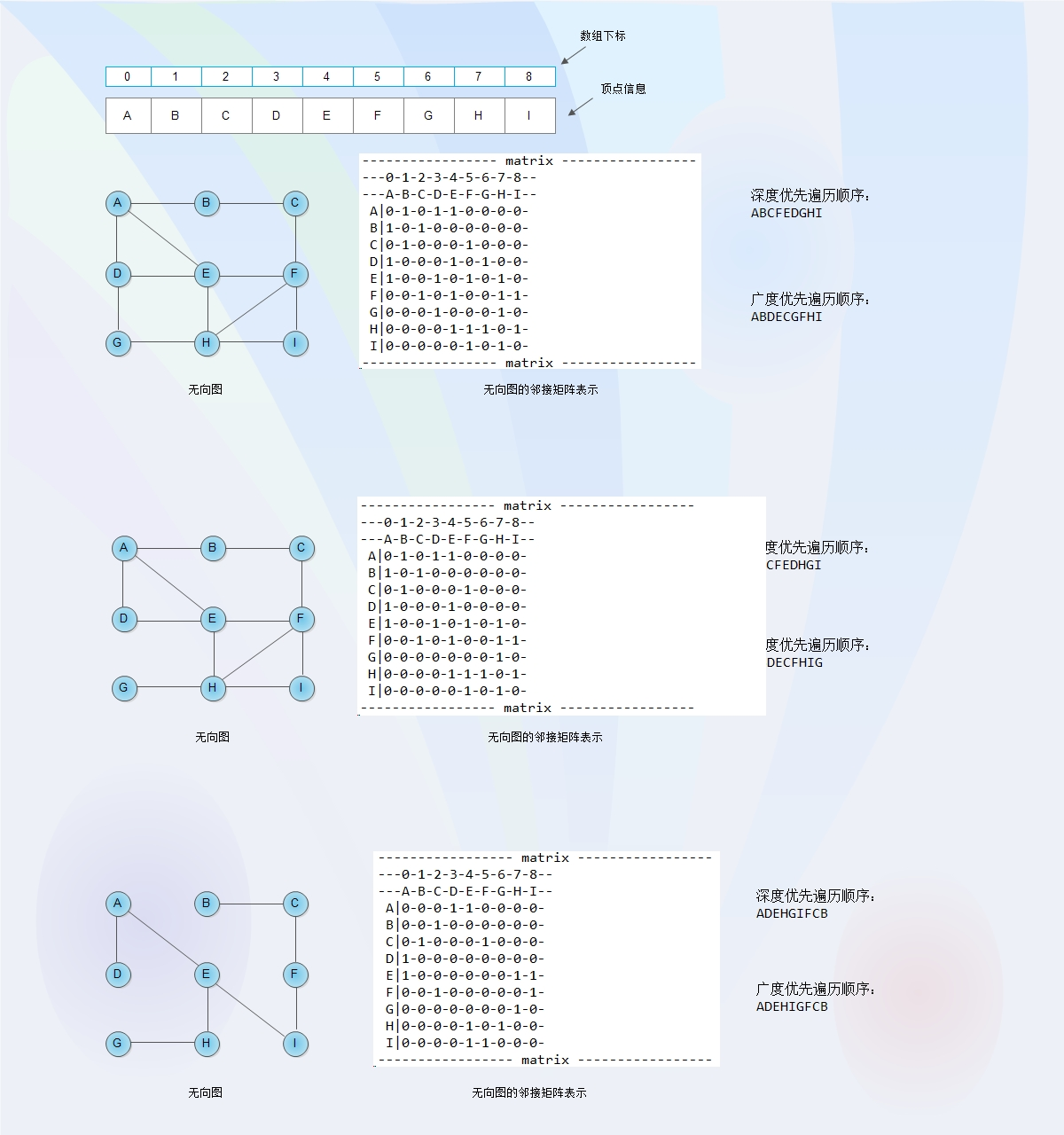深度和广度遍历代码如下：

package com.collonn.algorithm;

import java.util.Queue;
import java.util.Stack;

/**
* 无向图，邻接矩阵<br/>
* 深度优先遍历<br/>
* 广度优先遍历<br/>
*/
public class GrfDfsBfs {
private int total;
private String[] nodes;
private int[][] matirx;

public GrfDfsBfs(int total, String[] nodes) {
this.total = total;
this.nodes = nodes;
this.matirx = new int[total][total];
}

private void printMatrix() {
System.out.println("----------------- matrix -----------------");
System.out.println("---0-1-2-3-4-5-6-7-8--");
System.out.println("---A-B-C-D-E-F-G-H-I--");
for (int i = 0; i < this.total; i++) {
System.out.print(" " + this.nodes[i] + "|");
for (int j = 0; j < this.total; j++) {
System.out.print(this.matirx[i][j] + "-");
}
System.out.print("\n");
}
System.out.println("----------------- matrix -----------------");
}

// 设置[i][i]位置处的元素值为0，0表示图中的定点i未被访问，1表示图中的定点i已被访问
private void resetVisited() {
for (int i = 0; i < this.total; i++) {
this.matirx[i][i] = 0;
}
}

// 图的深度优先遍历(递归方法)
private void dfsRecursive(int i) {
// 如果已访问，则返回
if (this.matirx[i][i] == 1) {
return;
}

this.matirx[i][i] = 1;
System.out.print(this.nodes[i]);

for (int j = 0; j < this.total; j++) {
// i=j时，[i][j]表示节点是否被访问过，不参与dfs
if (i == j) {
continue;
}

if (this.matirx[i][j] == 1) {
dfsRecursive(j);
}
}
}

// 图的深度优先遍历(用栈实现)
private void dfsStack(Stack<Integer> stack) {
// 如果已访问，则返回
int k = -1;

while(!stack.empty()){
k = stack.peek().intValue();
boolean needPop = true;
for(int i = 0; i < this.total; i++){
if(this.matirx[k][i] == 1 && this.matirx[i][i] == 0){
stack.push(i);
this.matirx[i][i] = 1;
System.out.print(this.nodes[i]);
needPop = false;
break;
}
}
if(needPop){
stack.pop();
}
}
}

// 图的广度优先遍历
private void bfsQueue(Queue<Integer> ls) {
if (ls == null || ls.size() == 0) {
return;
}

int i = ls.poll().intValue();
// 如果已访问，则跳过该元素，继续访问队列的下一个元素
if (this.matirx[i][i] == 1) {
this.bfsQueue(ls);
return;
}

this.matirx[i][i] = 1;
System.out.print("" + this.nodes[i]);

for (int j = 0; j < this.total; j++) {
// i=j时，[i][j]表示节点是否被访问过，不参与bfs
if (i == j) {
continue;
}

//如果顶点已访问过,则不再加入队列
if (this.matirx[i][j] == 1 && this.matirx[j][j] != 1) {
ls.offer(j);
}
}

// System.out.print("\n队列元素:");
// for (Integer k : ls) {
// System.out.print(k);
// }
// System.out.println("");

this.bfsQueue(ls);
}

// 初始化图数据
// 0---1---2---3---4---5---6---7---8---
// A---B---C---D---E---F---G---H---I---
private void initGrf() {
// A-B, A-D, A-E
this.matirx = 1;
this.matirx = 1;
this.matirx = 1;
this.matirx = 1;
this.matirx = 1;
this.matirx = 1;
// B-C
this.matirx = 1;
this.matirx = 1;
// C-F
this.matirx = 1;
this.matirx = 1;
// D-E, D-G
this.matirx = 1;
this.matirx = 1;
this.matirx = 1;
this.matirx = 1;
// E-F, E-H
this.matirx = 1;
this.matirx = 1;
this.matirx = 1;
this.matirx = 1;
// F-H, F-I
this.matirx = 1;
this.matirx = 1;
this.matirx = 1;
this.matirx = 1;
// G-H
this.matirx = 1;
this.matirx = 1;
// H-I
this.matirx = 1;
this.matirx = 1;
}

// 初始化图数据
// 0---1---2---3---4---5---6---7---8---
// A---B---C---D---E---F---G---H---I---
private void initGrf2() {
// A-B, A-D, A-E
this.matirx = 1;
this.matirx = 1;
this.matirx = 1;
this.matirx = 1;
this.matirx = 1;
this.matirx = 1;
// B-C
this.matirx = 1;
this.matirx = 1;
// C-F
this.matirx = 1;
this.matirx = 1;
// D-E
this.matirx = 1;
this.matirx = 1;
// E-F, E-H
this.matirx = 1;
this.matirx = 1;
this.matirx = 1;
this.matirx = 1;
// F-H, F-I
this.matirx = 1;
this.matirx = 1;
this.matirx = 1;
this.matirx = 1;
// G-H
this.matirx = 1;
this.matirx = 1;
// H-I
this.matirx = 1;
this.matirx = 1;
}

// 初始化图数据
// 0---1---2---3---4---5---6---7---8---
// A---B---C---D---E---F---G---H---I---
private void initGrf3() {
// A-D, A-E
this.matirx = 1;
this.matirx = 1;
this.matirx = 1;
this.matirx = 1;
// B-C
this.matirx = 1;
this.matirx = 1;
// C-F
this.matirx = 1;
this.matirx = 1;
// E-H, E-I
this.matirx = 1;
this.matirx = 1;
this.matirx = 1;
this.matirx = 1;
// F-I
this.matirx = 1;
this.matirx = 1;
// G-H
this.matirx = 1;
this.matirx = 1;
}

public static void main(String[] args) {
String[] nodes = new String[] { "A", "B", "C", "D", "E", "F", "G", "H", "I" };
GrfDfsBfs grf = new GrfDfsBfs(9, nodes);
grf.initGrf();
grf.printMatrix();

System.out.println("------ 深度优先遍历(递归)开始 ------");
grf.resetVisited();
grf.dfsRecursive(0);
System.out.println();
System.out.println("------ 深度优先遍历(递归)结束 ------");

System.out.println("------ 深度优先遍历(栈)开始 ------");
grf.resetVisited();
Stack<Integer> stack = new Stack<Integer>();
stack.push(0);
grf.matirx = 1;
System.out.print(grf.nodes);
grf.dfsStack(stack);
System.out.println();
System.out.println("------ 深度优先遍历(栈)结束 ------");

System.out.println("------ 广度优先遍历开始 ------");
grf.resetVisited();
grf.bfsQueue(queue);
System.out.println();
System.out.println("------ 广度优先遍历结束 ------");
}

}

原创博文，转载请注明出处。

右键，查看图片，看大图。


展开全文广度优先遍历
• c语言实现的邻接表连通图深度优先遍历，包括的邻接表建立，深度优先遍历
• 主讲人 李 刚 深度优先遍历 C 目 录 ONTENTS 01 深度优先遍历实例演示 深度优先遍历实例演示 1 1 2 3 4 5 6 7 8 9 02 深度优先遍历实现过程 深度优先遍历实现过程 2 操作步骤 V1 V2 V5 V3 V0 V7 V6 V4 V8 在中...
• 17 、图深度优先遍历 编写程序对给定的有向（不一定连通）进行深度优先遍历中包含n个顶点，编号为0至n-1。本题限定在深度优先遍历过程中，如果同时出现多个待访问的顶点，则优先选择编号最小的一个进行访问，...
17 、图深度优先遍历
编写程序对给定的有向图（不一定连通）进行深度优先遍历，图中包含n个顶点，编号为0至n-1。本题限定在深度优先遍历过程中，如果同时出现多个待访问的顶点，则优先选择编号最小的一个进行访问，以顶点0为遍历起点。
输入格式:
输入第一行为两个整数n和e，分别表示图的顶点数和边数，其中n不超过20000，e不超过50。接下来e行表示每条边的信息，每行为两个整数a、b，表示该边的端点编号，但各边并非按端点编号顺序排列。
输出格式:
输出为一行整数，每个整数后一个空格，即该有向图的深度优先遍历结点序列。
输入样例1:
3 3
0 1
1 2
0 2
输出样例1:
0 1 2
输入样例2:
4 4
0 2
0 1
1 2
3 0
输出样例2:
0 1 2 3
代码如下：
import java.util.Scanner;

public class Main {
/** 存储节点信息*/
private int[] vertices;

/** 存储边信息（邻接矩阵）*/
private  int[][] arcs;

/** 图的节点数*/
private int vexnum;

/** 记录节点是否已被遍历*/
private boolean[] visited;

/** 初始化*/
public Main(int n) {
vexnum = n;
vertices = new int[n];
arcs = new int[n][n];
visited = new boolean[n];
for (int i = 0; i < vexnum; i++) {
for (int j = 0; j < vexnum; j++) {
arcs[i][j] = 0;
}
}
}

/** 添加边*/
public void addEdge(int i, int j){
if(i==j){
return ;
}
// 无向图对称的.
arcs[i][j]=1;
arcs[j][i]=1;
}

/** 设置节点集**/
public void setVertices(int[] vertices){
this.vertices=vertices;
}

/** 打印遍历节点*/
public void visit(int i){
System.out.print(vertices[i]+ " ");
}

/** 从第i节点开始深度优先遍历*/
public void traverse(int i){
// 标记第i个节点已遍历
visited[i]=true;
// 打印当前已经遍历的节点
visit(i);
// 遍历邻接矩阵中第i个节点的直接连通节点
for(int j=0;j<vexnum;j++){
if(arcs[i][j]==1&&visited[j]==false){
traverse(j);
}
}
}

public void dfs(){
// 初始化节点访问标记
for(int i=0;i<visited.length;i++){
visited[i]=false;
}
// 从没有被遍历的节点开始深度优先遍历
for(int i=0;i<vexnum;i++){
// 如果没有被访问过.
if(visited[i]==false){
traverse(i);
}
}
}
public static void main(String[] args) {
Scanner input=new Scanner(System.in);
int n=input.nextInt();
int e=input.nextInt();
Main dfs = new Main(n);
int[] vertices = new int[n];
// 添加节点集
for (int i=0;i<n;i++){
vertices[i]=i;
int a=input.nextInt();
int b=input.nextInt();
}
// 设置顶点集
dfs.setVertices(vertices);
dfs.dfs();
}
}

输出结果：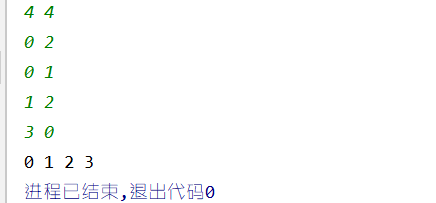展开全文• 图的深度优先遍历和广度优先遍历图的遍历深度优先遍历广度优先遍历 图的遍历 从给定图中任意指定的顶点（称为初始点）出发，按照某种搜索方法沿着图的边访问图中所有顶点，使每个顶点仅被访问一次，这个过程称为图的...广度优先
• 文章目录一、的概述1.1 什么是1.2 对比线性表和树1.3 的常见概念二、的存储方式2.1 邻接矩阵2.2 邻接表三、的遍历3.1 深度优先遍历3.1.1 什么是深度优先遍历3.1.2 深度优先遍历的步骤3.1.3 深度优先...广度优先遍历 深度搜索 广度搜索
• 【项目 - 迷宫问题之图深度优先遍历解法】 　设计一个程序，采用深度优先遍历算法的思路，解决迷宫问题。 　（1）建立迷宫对应的数据结构，并建立其邻接表表示。 　（2）采用深度优先遍历的思路设计算法，输出...数据结构 算法
• 深度优先遍历 广度优先遍历 的实现 深度优先遍历 广度优先遍历 的实现 深度优先遍历 广度优先遍历 的实现
• 深度优先遍历 （1）从中某个初始顶点v出发，首先访问初始顶点v。 （2）选择一个与顶点v相邻且没被访问过的顶点w，再从w出发进行深度优先搜索，直到中与当前顶点v邻接的所有顶点都被访问过为止。　 （3） 利用...数据结构 算法 链表
• 深度优先遍历 深度优先遍历参考 深度优先遍历 参考 1、https://blog.csdn.net/todd911/article/details/9191481 2、https://blog.csdn.net/weixin_42109012/article/details/94199335
• 邻接矩阵实现，Java实现数据结构，Java实现深度优先遍历，广度优先遍历java 算法 数据结构
• 创建有（无）向深度优先遍历，广度优先遍历 代码实现： #include<stdio.h> #include<stdlib.h> #include<string.h> #define MaxVertexNum 100 #define MaxSize 10 //顶点数目的最大值 ...
• 深度优先遍历连通图 从图中某个顶点v出发，访问v，并置visited[v]的值为true。 依次检查v 的所有邻接点w，如果visited[w]的值为false，再从w出发进行递归遍历，直至图中...{//从v个顶点出发递归地深度优先遍历图 ...
• 深度优先遍历和广度优先遍历深度优先遍历广度优先遍历 邻接表的形式表示一个： const graph = { 0: [1,2], 1: , 2: [3,0], 3: [3,0] } 深度优先遍历 深度优先遍历就是优先遍历完一条完整路径，和树的...数据结构
• 编写程序对给定的有向（不一定连通）进行深度优先遍历中包含n个顶点，编号为0至n-1。本题限定在深度优先遍历过程中，如果同时出现多个待访问的顶点，则优先选择编号最小的一个进行访问，以顶点0为遍历起点。 ...算法 链表 数据结构 dfs
• * 中节点值唯一标识这个节点 * */ public class graphTest { static Map<Integer,LinkedList<Integer>> undirectedGraph = new LinkedHashMap<>(); static Map<Int...
• //从顶点v出发，广度优先遍历图G，算法借助一个辅助队列 void BFS(ALGraph G,int v){ visit(v); visited[v]=true; Enqueue(Q,v); while(!Empty(Q)){ DeQueue(Q,v); for(p=G.vertices[v].firstarc;p;p=p->...
• 主要介绍了python实现树的深度优先遍历与广度优先遍历,详细分析了树的深度优先遍历与广度优先遍历原理及Python相关实现技巧,需要的朋友可以参考下
• 主要介绍了JavaScript树的深度优先遍历和广度优先遍历算法,结合实例形式分析了JavaScript树的深度优先遍历、广度优先遍历递归与非递归相关实现技巧,需要的朋友可以参考下
•广度优先遍历
• （3）若此时中尚有顶点未被访问，则从一个未被访问的顶点出发，重新进行深度优先遍历，直到中所有顶点均被访问过为止。 递归实现深度遍历 通过递归实现深度优先遍历 let depth1 = (node, nodeList = []) => ...广度优先遍历 js 遍历
• 深度优先遍历深度优先遍历是图论中的经典算法。其利用了深度优先搜索算法可以产生目标的相应拓扑排序表，采用拓扑排序表可以解决很多相关的图论问题，如最大路径问题等等。 根据深度优先遍历的特点我们利用Java...广度优先遍历...